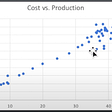# Factor Analysis in Red Wine Quality

## Why using Factor Analysis?

The general purposes of doing factor analysis (FA) is to simplify the data by reducing various variables into smaller dimensions. This technique combines all of the variables’ largest common variance into a single outcome. In this article, FA is used to reduce variables (such as free sulfur dioxide and total sulfur dioxide) in the red quality wine dataset by combining them into one variable/dimension to reduce the amount of time and money spent on calculation/analysis.

## Why Non-Metric Independent Variable was Excluded from FA?

FA uses Pearson correlation to assess the similarity between each pair of variables, and Pearson correlation is a numerical value that can only be calculated using metric variables, while non-metric independent variables were not included in the analysis.

# Factor Analysis Outputs

Based on rotated component matrix figure, the independent metric variables can be grouped into four factors, namely:

• Factor 1: Fixed Acidity, Density, Citric Acid, pH
• Factor 2: Quality, Alcohol, Volatile Acidity
• Factor 3: Free Sulfur Dioxide, Total Sulfur Dioxide, Residual Sugar
• Factor 4: Chlorides, Sulphates

Of the four factors above, factor 1 can be categorized as “Acid and Density” based on the variables contained in these factors. In addition, factor 2 can be categorized as “Alcohol and Quality” while factor 3 is “Sulfur and Residual Sugar”. Moreover, factor 4 can be categorized as “Chemicals”.

## Communality

The percentage of variance accounted for by the components identified through FA is referred to as communality. In communalities figure shows that if the quality is predicted using the four variables obtained from FA by MLR, the R^2 value of the model is 0.627.

## Eigenvalue

The eigenvalue may be described as each component’s quality score; factors with high eigenvalues are more likely to reflect the underlying factor. As a general guideline, factors with an eigenvalue of 1 or above should be used. In this scenario, as shown in eigenvalues scree plot and component matrix figure, the dataset’s 12 variables assess four underlying components. Therefore, four factors are chosen.

# Evaluating outputs

## Increase the factorability

KMO-MSA, as shown KMO and Bartlett’s test output, may be used to determine the dataset’s factorability. Because MSA is less than 0.5, the dataset is not factorable. However, to make the dataset factorable, it is necessary to deletion variables that have a Pearson correlation close to 0 (in this case, deletion is carried out on the residual sugar variable). After deletion of the residual sugar variable, the following is the new FA output:

There is no cross-loading for any of the variables, as shown in new rotated component matrix table. Despite the fact that the density variable has two values in two separate components, factor 1 has a considerably higher density value than factor 2. If the dataset is cross-loaded, the problem can be solved by using a rotation technique. If the cross-loading is continuous, the variable should be removed and FA recalculated.

If the dataset is having cross-loading issue, the problem can be solved by using a rotation technique to minimize the cross-loading issue. Cross-loading is intended to be reduced by using Varimax with the Kaiser Normalization method of rotation. However, if the cross-loading is continuous, the variable should be removed and FA recalculated.

# References

The SPSS file and outputs are available on my GitHub here.

--

--

--

## More from Mario Caesar

Hello World! 👋 | Just Nobody on Medium | linktr.ee/caesarmario_

Love podcasts or audiobooks? Learn on the go with our new app.

## Simple Linear Regression## Intro to Monte Carlo Simulation Using Business Examples## Are Stock Returns Normally Distributed?## Baffled by Elasticity? use it to set the right price for your product.## The 6 Trends You Should Know About Enterprise Data Innovation## Web Scraping with Python Made Easy## Case Study: Find The Cheapest Rooms With High-Score Ratings (Part 2)## Mario Caesar

Hello World! 👋 | Just Nobody on Medium | linktr.ee/caesarmario_

## Water Quality Analysis## Introduction to the Measures of Central Tendency and Dispersion## Multiple Linear Regression in Red Wine Quality## Data science metrics trap : McNamara Fallacy